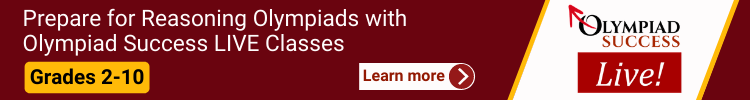All fields are required

The CREST Reasoning Olympiad is held at a single level:
Level 1: The respective school is the venue for students appearing through schools. For those students who are registered as an individual will have to take exam from home or from institute having computer with good internet connectivity.
The Level 1 exam is an objective-type test having duration of 60 minutes comprising of 35 objective-type questions for classes 1 to 4 and 50 objective-type questions for classes 5 to 10.
The exam consists of two sections:

• Section-1: Logical Reasoning
• Section-2: Achievers SectionOther Important Info:

• There are separate question papers for each and every class.
• The medium of the exam is English.
• CBSE, ICSE/ISC and other State Board syllabus is followed for the setting of test papers.
• The exam is conducted during school hours only
Class 1

Section 1: Analogy, Geometrical Shapes, Grouping of Figures, Embedded Figures, Odd One Out, Pattern, Measuring Units, Ranking Test, Spatial Understanding.

Achievers Section: Higher Order Thinking Questions - Syllabus as per Section 1

Class 2

Section 1: Analogy, Coding and Decoding, Geometrical Shapes, Embedded Figures, Odd One Out, Pattern, Measuring Units, Grouping of Figures, Ranking Test, Series Completion.

Achievers Section: Higher Order Thinking Questions - Syllabus as per Section 1

Class 3

Section 1: Patterns, Analogy and Classification, Geometrical Shapes, Mirror Images and Water Images, Direction Sense Test, Ranking Test, Alphabet Test and Logical Sequence of Words, Puzzle Test, Coding - Decoding.

Achievers Section: Higher Order Thinking Questions - Syllabus as per Section 1

Class 4

Section 1: Patterns, Analogy and Classification, Geometrical Shapes and Solids, Embedded Figures, Mirror Images and Water Images, Direction Sense Test, Possible Combinations, Ranking Test, Alphabet Test and Logical Sequence of Words, Puzzle Test, Coding - Decoding.

Achievers Section: Higher Order Thinking Questions - Syllabus as per Section 1

Class 5

Section 1: Patterns, Analogy and Classification, Geometrical Shapes and Solids, Embedded Figures, Mirror Images and Water Images, Direction Sense Test, Possible Combinations, Ranking Test, Alphabet Test and Logical Sequence of Words, Puzzle Test, Coding - Decoding.

Achievers Section: Higher Order Thinking Questions - Syllabus as per Section 1

Class 6

Section 1: Series Completion and Inserting the Missing Character, Analogy and Classification, Blood Relations, Coding - Decoding, Direction Sense Test, Logical Venn Diagram, Alpha Numeric Sequence Puzzle, Number, Ranking and Time Sequence Test, Logical Sequence of Words, Alphabet Test, Mathematical Operations, Analytical Reasoning, Mirror Images and Water Images, Embedded Figures, Figure Formation, Construction of Squares, Grouping of Identical Figures, Figure Matrix, Paper Folding and Paper Cutting, Cubes and Dice, Dot Situations.

Achievers Section: Higher Order Thinking Questions - Syllabus as per Section 1

Class 7

Section 1: Series Completion and Inserting the Missing Character, Analogy and Classification, Blood Relations, Coding - Decoding, Direction Sense Test, Logical Venn Diagram, Alpha Numeric Sequence Puzzle, Number, Ranking and Time Sequence Test, Logical Sequence of Words, Alphabet Test, Mathematical Operations, Analytical Reasoning, Mirror Images and Water Images, Embedded Figures, Figure Formation, Construction of Squares, Grouping of Identical Figures, Figure Matrix, Paper Folding and Paper Cutting, Cubes and Dice, Dot Situations.

Achievers Section: Higher Order Thinking Questions - Syllabus as per Section 1

Class 8

Section 1: Series Completion and Inserting the Missing Character, Analogy and Classification, Blood Relations, Coding-Decoding, Direction Sense Test, Logical Venn Diagram, Alpha Numeric Sequence Puzzle, Number, Ranking and Time Sequence Test, Logical Sequence of Words, Alphabet Test, Mathematical Operations, Analytical Reasoning, Mirror Images and Water Images, Embedded Figures, Figure Formation, Construction of Squares, Grouping of Identical Figures, Figure Matrix, Paper Folding and Paper Cutting, Cubes and Dice, Dot Situations.

Achievers Section: Higher Order Thinking Questions - Syllabus as per Section 1

Class 9

Section 1: Series Completion, Analogy and Classification, Coding-Decoding, Blood Relations, Puzzle Test, Sequential Output Tracing , Direction Sense Test, Logical Venn Diagrams, Alphabet Test and Logical, Alpha-Numeric, Number, Mathematical Operations, Inserting the Missing Characters, Analytical Reasoning, Mirror Images and Water Images, Spotting Out The Embedded, Figure Matrix, Paper Folding and Paper Cutting, Grouping of Identical Figures, Cubes and Dice, Dot Situation, Construction of Squares and Triangles, Figure Formation and Analysis.

Achievers Section: Higher Order Thinking Questions - Syllabus as per Section 1

Class 10

Section 1: Series Completion, Analogy and Classification, Coding - Decoding, Blood Relations, Sequential Output Tracing , Direction Sense Test, Logical Venn Diagrams, Alphabet Test and Logical, Alpha-Numeric, Number, Mathematical Operations, Inserting the Missing Characters, Analytical Reasoning, Mirror Images and Water Images, Spotting Out The Embedded, Figure Matrix, Paper Folding and Paper Cutting, Grouping of Identical Figures, Cubes and Dice, Dot Situation, Construction of Squares and Triangles, Figure Formation and Analysis.

Achievers Section: Higher Order Thinking Questions - Syllabus as per Section 1